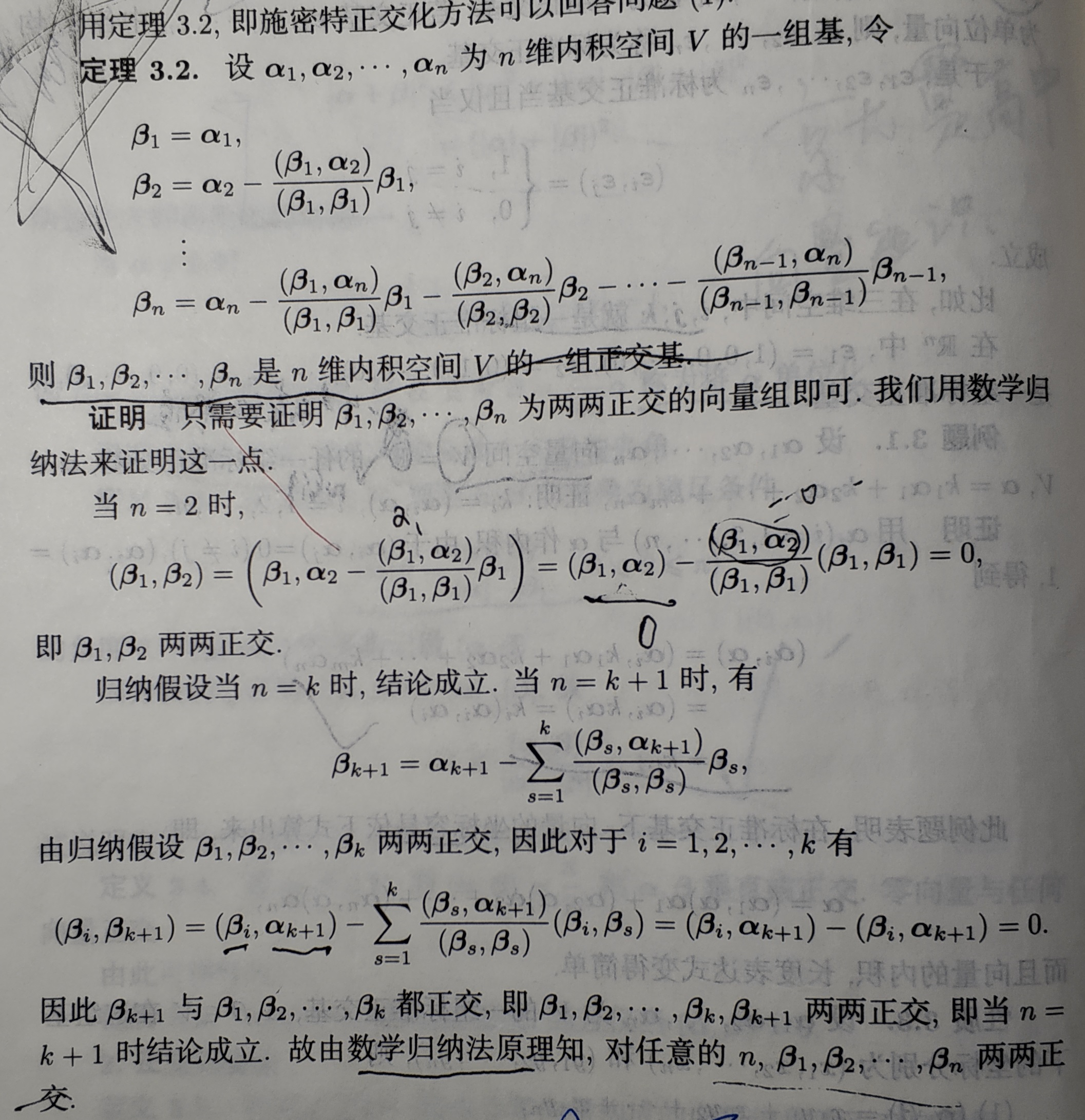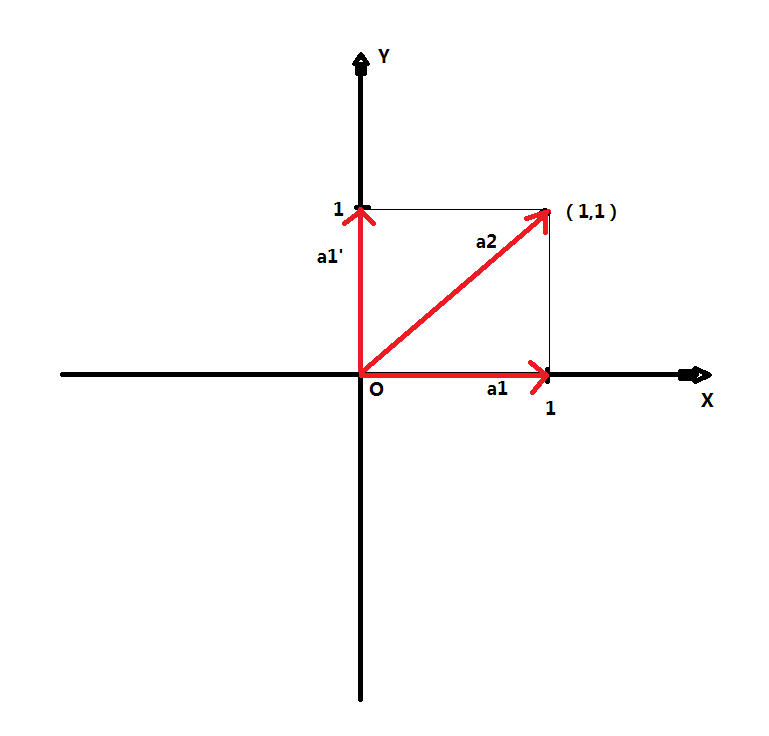# 线性代数中一组基向量的标准正交化原理通熟易懂理解

13 篇文章 2 订阅1.首先我们看看这个正交化过程，因为a1,a2...an为一组基向量（大佬们请原谅我用a字母代替阿尔法希腊字母，实在是太难输入了，嘻嘻！），只是满足k1a1+k2a2+k3a3+...knan = 0时候，一定有系数k1=k2=...=kn=0，但是如果，a1,a2...an没有任意两者内积为零，也就是他们不是正交的，这就说明了一个问题，a1,a2...an这些基向量之间有冗余（如果是正交基的话，应该是不存在这个问题的），意思就是他们之间的一些分量可能重叠了，还不理解?（我以前学习的时候听到这些也是一脸蒙逼）哈哈，没关系，那我就来举个例子吧，我们看下面的例子：

a1 = (1,0) , a2 = (0,1)

k1a1+k2a2 = 0,看看是否k1=k2=0,如果k1=k2=0,那么a1,a2线性无关，否则就是线性相关了

k1(1,0)+k2(0,1) = 0，很明显了，k1=k2=0，所以a1,a2线性无关，而且a1*a2 = 0，（这里面*都表示向量内积）所以a1垂直a2，即a1,a2正交，那么说明a1,a2是一组正交基，

a1 = (1,0)，a2 = (1,1)

（不写了，凌晨1.24了，已经很晚了，以前高中为了考个牛逼点的985，那时候经常一点多才睡，嘻嘻！不过现在不想那样了，得赶紧睡觉，明天早上继续写，晚安各位大佬！）a2 = c*e1 + a1'，（注：因为我们已经令e1=a1/|a1|，即e1已经是单位化的了，所以a2在a1向量方向上的单位分量肯定得带一个系数c，刚好我举的这个例子这里c=1罢了），现在我们只要能找出这个a1’向量（因为前面没带系数，那么我们找到的a1‘向量可能不是单位化的，我们一定得正交化一次，才能成为第二个标准正交基）即可，

a1' = a2 - c*e1 即为我们所求，这里因为c是未知系数，我们是求不出a1’的，因此我们得先求出系数c即可

（a2 - c*e1）*e1 =0 ，展开得到

a2*e1 = c*e1*e1，因为e1*e1 = 1（单位向量与自己内积当然是等于模长啦！就是=1的）即

c = a2*e1 = (a2,e1) （写成了向量内积的括号表示方式）

a1' = a2 - (a2,e1)*e1 = a2 - e1 = （0,1）

β1 = a1，（我刚刚标准正交基向量用的是e1，e2的符号，书上这里用的是β1，β2，不过没关系，知道就好）

β2 = a2 - （β1，a2） / （β1，β1） * β1

β2 = （1,1）- 1/1 * （1,0） = （0,1）

（h2，e1） = （g2，e1）- （c21*e1，e1） = 0

c21 = （g2，e1），即可求得上面的h2

c31 = （g3，e1），c32 = （g3，e2），即可求得h3

cn1 = （gn，e1），cn2 = （gn，e2），cn3 = （gn，e3）.....cn(n-1) = (gn，e（n-1））

h2 = g2 - c21*e1，求出c21，利用条件h2⊥e1，即（h2，e1） = （g2，e1）- （c21*e1，e1） = 0

（an，A） = an*cn*an = cn*1 = cn 这个系数了嘛（an*an = 1的，因为是标准正交基，模长为1），上面红色加粗字体部分我们求向量的系数是如此，实际上求函数的标准正交基的系数也是如此，下面我举一个函数的例子：

f（x） = a0*1/π*cos0x + b0*1/π*sin0x(注：这一项=0) + a1*1/π*cosx + b1*1/π*sinx + a2 * 1/π*cos2x + b2*1/π*sin2x + ...... + an*1/π*cosnx + bn*1/π*sinnx

f（x） = a0*1/π + a1*1/π*cosx + b1*1/π*sinx + a2 * 1/π*cos2x + b2*1/π*sin2x + ...... + an*1/π*cosnx + bn*1/π*sinnx）

a1 = (1/π*cosx，f(x)）= ∫f(x)*1/π*cosxdx，                b1 = (1/π*sinx，f(x) ）= ∫f(x)*1/π*sinxdx

a2 = (1/π*cos2x，f(x)）= ∫f(x)*1/π*cos2xdx，            b2 = (1/π*sin2x，f(x)）= ∫f(x)*1/π*sin2xdx

......

an = (1/π*cosnx，f(x)）= ∫f(x)*1/π*cosnxdx，            bn = (1/π*sinnx，f(x)）= ∫f(x)*1/π*sinnxdx

an = 1/π*∫f(x) * cosnxdx       (n =0,1,2,3....)，特别的，当n=0时，a0 = 1/π∫f(x)dx

bn = 1/π*∫f(x) * sinnxdx        (n =1,2,3....)

03-142396
05-24143807-098086
07-139606
08-144051
12-062万+
08-136312
12-171339
03-241287
09-042026
07-041万+
12-091056
08-212805
08-232848
11-201万+
09-091万+
12-027981
04-171万+
10-221万+点击重新获取扫码支付余额充值